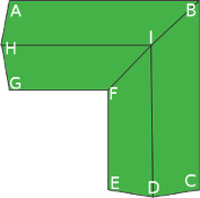-

# Cross Gabled

No pitch required (how )* This image is to clarify which is the rise of the roof (H) and the rise of the dormer (h). The image is for general informational purpose.
3D

L1
L2
l1
H
* L is the length of the roof
* l is the width of the roof
* H is the rise (height) of the roof (3D image)
* For decimal dimensions, use the dot notation! (.)

### Total Roof area:

...

#### Roof Lengths

AH = HG = CD = DE = ...
BI = FI = ...
AB = ...
BC = ...
EF = ...
GF = ...
HI = ...
ID = ...ABIH = ...
BCDI = ...
DEFI = ...
FGHI = ...

#### Roof Slopes

ABIH = BCDI = DEFI = FGHI
• Slope Grade = ... %
• Angle = ... deg
• Pitch = ... x12* This image is to clarify which is the rise of the roof (H) and the rise of the dormer (h). The image is for general informational purpose.
3D

L1
L2
l1
H
Small dormer:
Lu 0
Lu 1
h
* L is the length of the roof
* l is the width of the roof
* H is the rise (height) of the roof (3D image)
* For decimal dimensions, use the dot notation! (.)

### Total Roof area:

...

#### Roof Lengths

AH = HG = CD = DE = ...
BI = FI = ...
AB = ...
BC = ...
EF = ...
GF = ...
HI = ...
ID = ...ABIH = ...
BCDI = ...
DEFI = ...
FGHI = ...

#### Roof Slopes

ABIH = BCDI = DEFI = FGHI
• Slope Grade = ... %
• Angle = ... deg
• Pitch = ... x12* This image is to clarify which is the rise of the roof (H) and the rise of the dormer (h). The image is for general informational purpose.
3D

L1
L2
l1
H
Large dormer:
Lu 0
Lu 1
Lu 2
h
* L is the length of the roof
* l is the width of the roof
* H is the rise (height) of the roof (3D image)
* For decimal dimensions, use the dot notation! (.)

### Total Roof area:

...

#### Roof Lengths

AH = HG = CD = DE = ...
BI = FI = ...
AB = ...
BC = ...
EF = ...
GF = ...
HI = ...
ID = ...ABIH = ...
BCDI = ...
DEFI = ...
FGHI = ...

#### Roof Slopes

ABIH = BCDI = DEFI = FGHI
• Slope Grade = ... %
• Angle = ... deg
• Pitch = ... x12* This image is to clarify which is the rise of the roof (H) and the rise of the dormer (h). The image is for general informational purpose.
3D

L1
L2
l1
H
Small dormer:
Lu 0
Lu 1
h
Small dormer 2:
Lu 3
Lu 4
h 2
* L is the length of the roof
* l is the width of the roof
* H is the rise (height) of the roof (3D image)
* For decimal dimensions, use the dot notation! (.)

### Total Roof area:

...

#### Roof Lengths

AH = HG = CD = DE = ...
BI = FI = ...
AB = ...
BC = ...
EF = ...
GF = ...
HI = ...
ID = ...ABIH = ...
BCDI = ...
DEFI = ...
FGHI = ...

#### Roof Slopes

ABIH = BCDI = DEFI = FGHI
• Slope Grade = ... %
• Angle = ... deg
• Pitch = ... x12* This image is to clarify which is the rise of the roof (H) and the rise of the dormer (h). The image is for general informational purpose.
3D

L1
L2
l1
H
Large dormer:
Lu 0
Lu 1
Lu 2
h
Large dormer 2:
Lu 4
Lu 5
Lu 6
h 2
* L is the length of the roof
* l is the width of the roof
* H is the rise (height) of the roof (3D image)
* For decimal dimensions, use the dot notation! (.)

### Total Roof area:

...

#### Roof Lengths

AH = HG = CD = DE = ...
BI = FI = ...
AB = ...
BC = ...
EF = ...
GF = ...
HI = ...
ID = ...ABIH = ...
BCDI = ...
DEFI = ...
FGHI = ...

#### Roof Slopes

ABIH = BCDI = DEFI = FGHI
• Slope Grade = ... %
• Angle = ... deg
• Pitch = ... x12* This image is to clarify which is the rise of the roof (H) and the rise of the dormer (h). The image is for general informational purpose.
3D

L1
L2
l1
H
Large dormer:
Lu 0
Lu 1
Lu 2
h
Small dormer:
Lu 4
Lu 5
h 2
* L is the length of the roof
* l is the width of the roof
* H is the rise (height) of the roof (3D image)
* For decimal dimensions, use the dot notation! (.)

### Total Roof area:

...

#### Roof Lengths

AH = HG = CD = DE = ...
BI = FI = ...
AB = ...
BC = ...
EF = ...
GF = ...
HI = ...
ID = ...ABIH = ...
BCDI = ...
DEFI = ...
FGHI = ...

#### Roof Slopes

ABIH = BCDI = DEFI = FGHI
• Slope Grade = ... %
• Angle = ... deg
• Pitch = ... x12